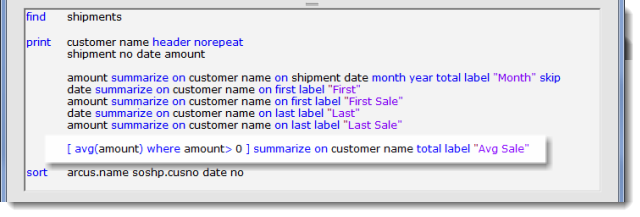# Print — Summarize

### Expression summarize

The target field can be a dictionary or in-line expression. To our example we will add an in-line expression of the average shipment amount:• [ avg(amount) where amount > 0 ]
• avg(amount)avg is a special reserved word that will compute the average
• where — like the main where clause, this introduces the conditions for the field
• amount > 0 — the condition is that the shipment amount is greater than zero.
• [ and ] — brackets define the in-line expression.

The last column is now the average Sale for each customer:Page 1 — summarizePage 2 — summarize syntaxPage 3 — numeric summarizePage 4 — date summarizePage 5 — expression summarizePage 6 — text summarize

Next – text summarize >